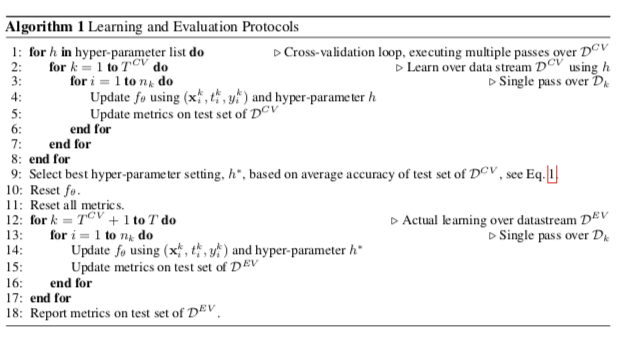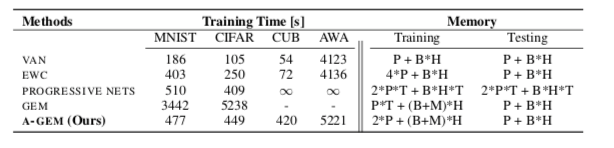# Efficient lifelong learning with A-GEM

## Learning protocol

$D^{CV}={D1,…,D{T^{CV}}}$ 和 $D^{EV} = {D_{T^{CV}+1},…,D_T}$

$D^{CV}$是交叉验证期间将使用的数据集的流。$D^{CV}$允许学习者出于模型超参数选择的目的多次重放所有样本。## Metrics

### Average Accuracy

$A\in [0,1]$ 用所有小批量连续训练模型后的平均精度，直到任务k被定义为：

$A_T$ 是所有任务的平均准确率，是最后一个任务学习后得到的。这是LLL中最常用的度量。

### Forgetting Measure

$F \in [-1,1]$ 模型经过所有小批量连续训练后的平均遗忘，直到任务 k 被定义为：

### Learning Curve Area

$LCA \in [0,1]$ 让我们首先定义模型在所有 T 任务训练后的平均 b-shot 性能（其中 b 是小批量数）：

$\beta$ 处的 LCA 是作为 $b \in [0, \beta]$ 函数的收敛曲线 $Z_b$ 的面积：

LCA 有一个直观的解释。 $LCA0$ 是平均 0-shot 表现，与 GEM 中的前向转移相同。 $LCA{\beta}$ 是 $Zb$ 曲线下的区域，如果 0-shot 性能好并且学习器学习速度快，则该区域很高。 特别是，可能有两个模型具有相同的 $Z_b$ 或 $A_T$ ，但 $LCA{\beta}$ 非常不同，因为一个模型比另一个学习得快得多，而它们最终都获得了相同的最终精度。 该度量旨在区分这两种情况，并且对于相对较小的 $\beta$ 值是有意义的，因为我们对从少数示例中学习的模型感兴趣。

## Averaged gradient episodic memory (A-GEM)

A-GEM 建立在 GEM 的基础上，该算法利用小的情节记忆在单遍设置中表现良好，并对损失函数提出了一个小的改变，使 GEM 在 训练时间，同时保持相似的表现；

### 回顾GEM

GEM在计算和内存成本方面很高。GEM通过为每个任务 $k$ 存储情节记忆 $M_k$ 来避免灾难性遗忘。### A-GEM

GEM 确保在每个训练步骤中，每个单独的先前任务的损失（由情景记忆中的样本近似）不会增加

• 1）不需要计算矩阵 G 而只需要计算内存样本的随机子集的梯度

• 2）它不需要解决任何 QP，只需要解决一个内积

• 3）它会产生更少的违背约束，特别是当任务数量很大时。

所有这些因素一起使 A-GEM 更快，同时不会妨碍其在单程设置中的良好性能。

A-GEM的优化目标: